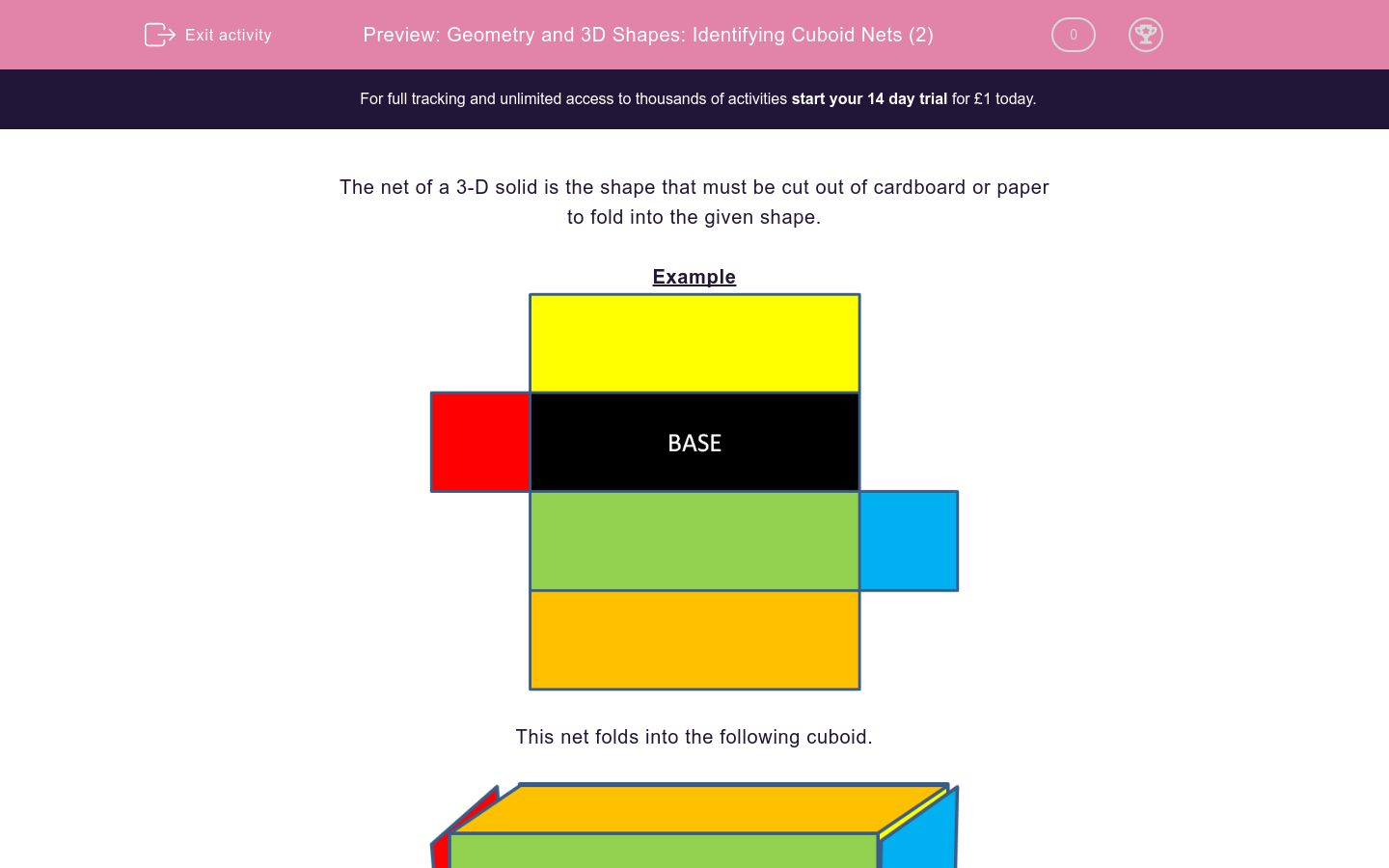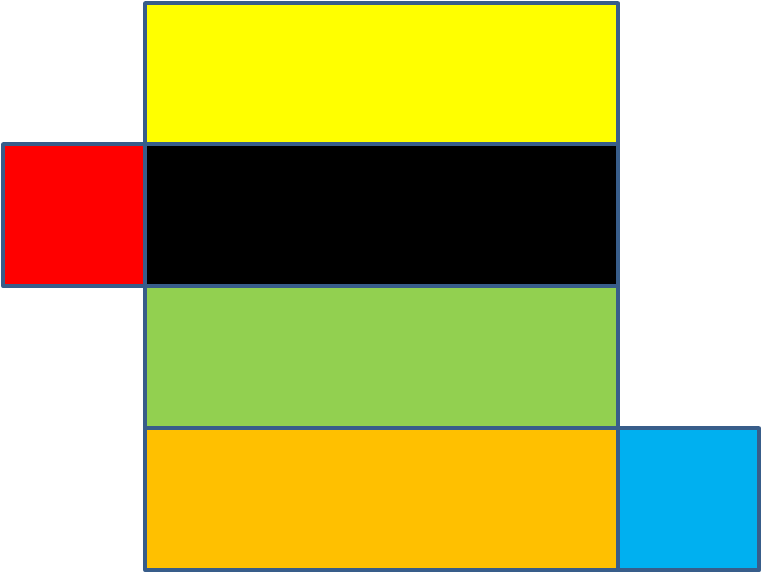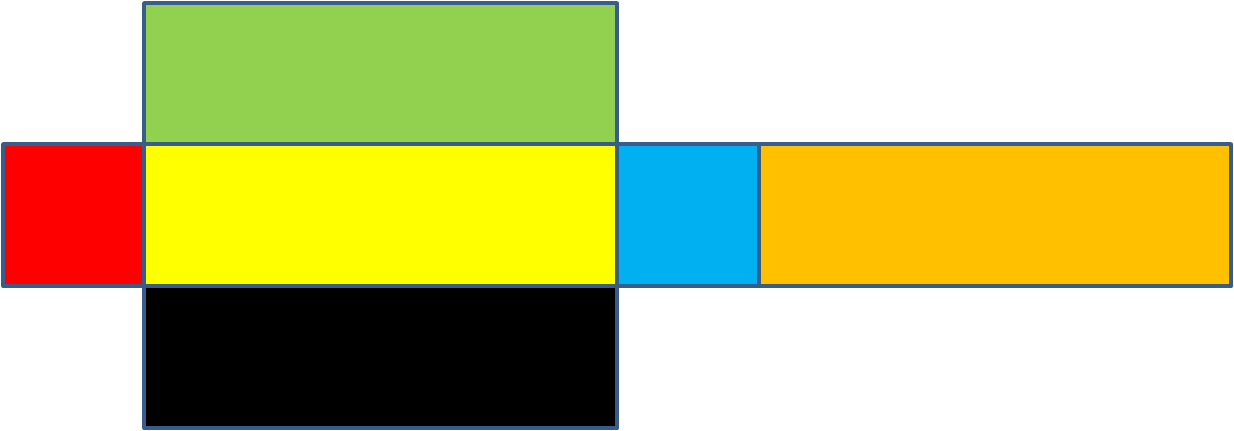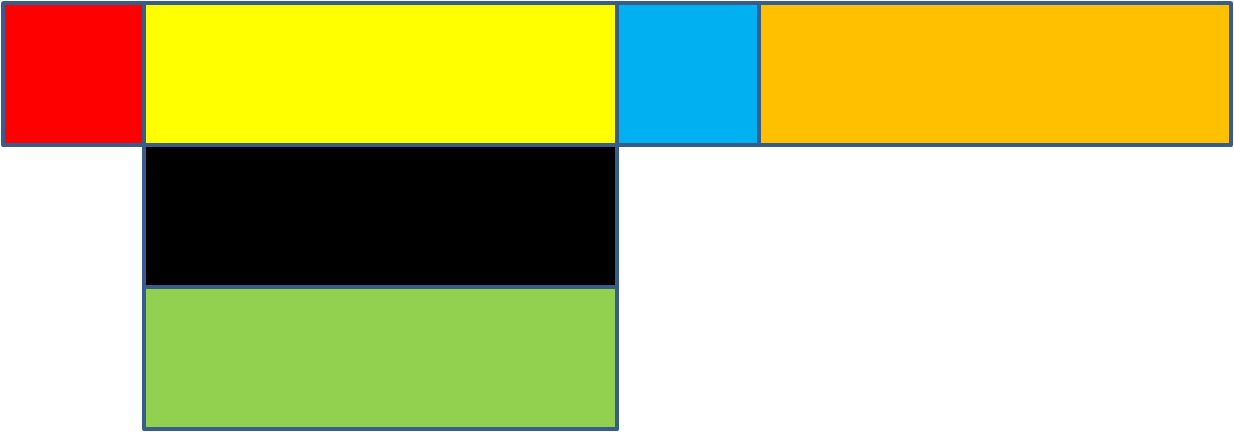# Geometry and 3D Shapes: Identifying Cuboid Nets (2)

In this worksheet, students identify nets of cuboids.Key stage:  KS 2

Curriculum topic:  Maths and Numerical Reasoning

Curriculum subtopic:  3D Shapes

Difficulty level:### QUESTION 1 of 10

The net of a 3-D solid is the shape that must be cut out of cardboard or paper

to fold into the given shape.

ExampleThis net folds into the following cuboid.True or False?

This is a net of a cuboid.True

False

True or False?

This is a net of a cuboid.True

False

True or False?

This is a net of a cuboid.True

False

True or False?

This is a net of a cuboid.True

False

True or False?

This is a net of a cuboid.True

False

True or False?

This is a net of a cuboid.True

False

True or False?

This is a net of a cuboid.True

False

True or False?

This is a net of a cuboid.True

False

True or False?

This is a net of a cuboid.True

False

True or False?

This is a net of a cuboid.True

False

• Question 1

True or False?

This is a net of a cuboid.True
• Question 2

True or False?

This is a net of a cuboid.True
• Question 3

True or False?

This is a net of a cuboid.True
• Question 4

True or False?

This is a net of a cuboid.False
• Question 5

True or False?

This is a net of a cuboid.False
• Question 6

True or False?

This is a net of a cuboid.True
• Question 7

True or False?

This is a net of a cuboid.False
• Question 8

True or False?

This is a net of a cuboid.True
• Question 9

True or False?

This is a net of a cuboid.True
• Question 10

True or False?

This is a net of a cuboid.True
---- OR ----

Sign up for a £1 trial so you can track and measure your child's progress on this activity.

### What is EdPlace?

We're your National Curriculum aligned online education content provider helping each child succeed in English, maths and science from year 1 to GCSE. With an EdPlace account you’ll be able to track and measure progress, helping each child achieve their best. We build confidence and attainment by personalising each child’s learning at a level that suits them.

Get startedStart your £1 trial today.
Subscribe from £10/month.